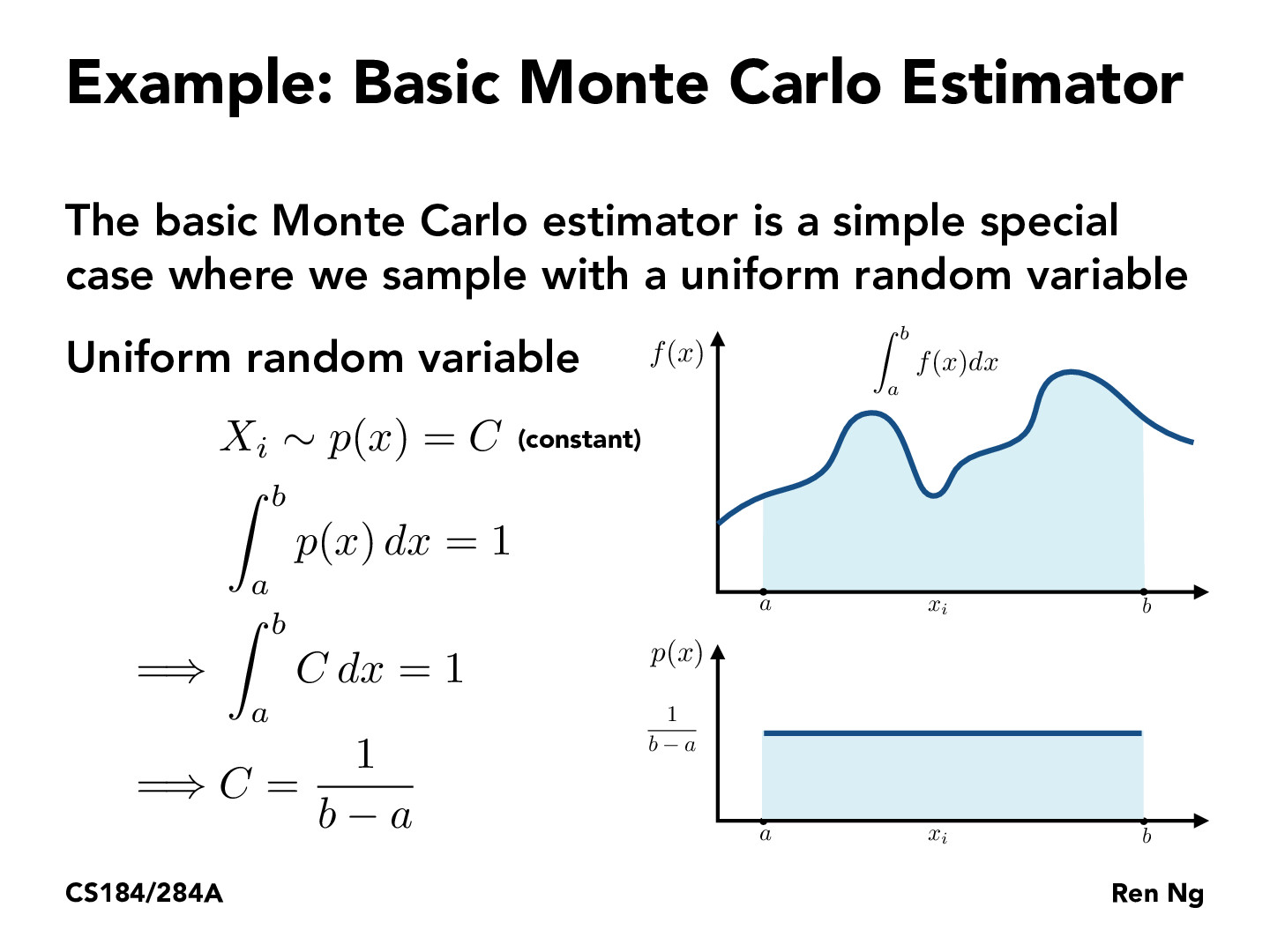Lecture 12: Monte Carlo Integration (22)yao12310

In what cases might one use non-uniform random variables? It seems like the fact that the estimate is unbiased is independent of the distribution used, so wondering how one can decide on a superior distribution to the uniform.rileylyman184

Suppose we take the integral of f(x) over the range (a,b). This will get us some value A. Now suppose we divide A by the length of the interval, (b-a). We know that the function A/(b-a) and f(x) along the range (a,b) both have the same value. For the former case, we can calculate the area as just the area of the rectangle of height A/(b-a) and width (b-a), which turns out to be A. Then, for the integral, we get A as before, showing that they are the same.

So, A/(b-a) is just the true average value of f(x) along the interval (a,b). Note that for our basic monte carlo estimator, we are summing 1/N times the sum of f(x_i) for N such x_i. The greater N becomes, the closer this sum gets to the average value of f(x), which is A/(b-a). That is why is makes sense that we multiply this result by (b-a) to get the final monte carlo estimator, since this gets us very close to the real integral value A for large N.

You must be enrolled in the course to comment